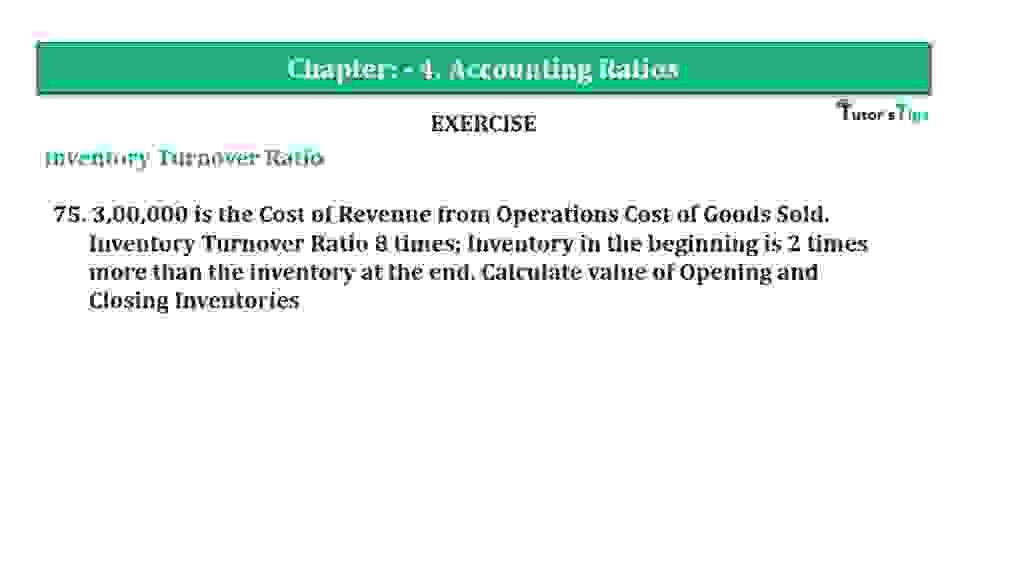# Question 75 Chapter 4 of +2-B – T.S. Grewal 12 ClassQuestion 75 Chapter 4 of +2-B

Interest Coverage Ratio

75. 3,00,000 is the Cost of Revenue from Operations Cost of Goods Sold.
Inventory Turnover Ratio 8 times; Inventory, in the beginning, is 2 times more than the inventory at the end. Calculate the value of Opening and Closing Inventories

### The solution of Question 75 Chapter 4 of +2-B: –

 Inventory Turnover Ratio = Cost of goods sold Average Inventory
 8 = Rs. 3,00,000 Average Inventory Average Inventory = Rs. 3,00,000 / 8 Average Inventory = Rs. 37,500 Opening Inventory = 2x + x Closing Inventory = X

 Average Inventory = Opening Inventory + Closing Inventory 2
 Rs. 37,500 = (2x + X) + X 2 4x = Rs. 75,000

 X = Rs. 75,500 4 = Rs. 18,750

 Opening Inventory = Rs. 18,750 Closing Inventory = 3 x Rs. 18,750 = Rs. 56,250

Balance Sheet: Meaning, Format & Examples

Thanks, Please Like and share with your friends

Comment if you have any question.

Also, Check out the solved question of previous Chapters: –

### T.S. Grewal’s Double Entry Book Keeping (Vol. II: Accounting for Companies)T.S. Grewal’s Analysis of Financial Statements